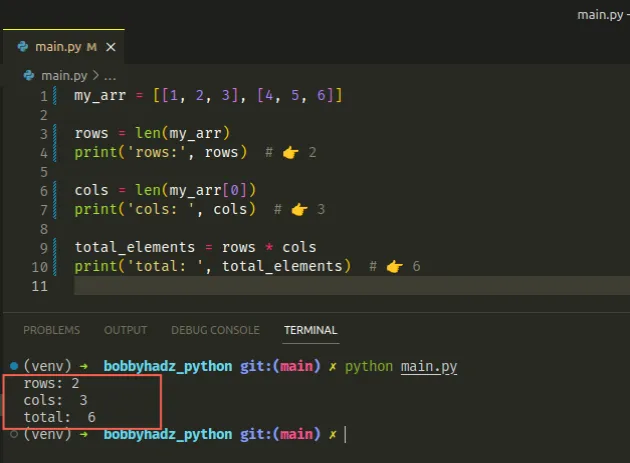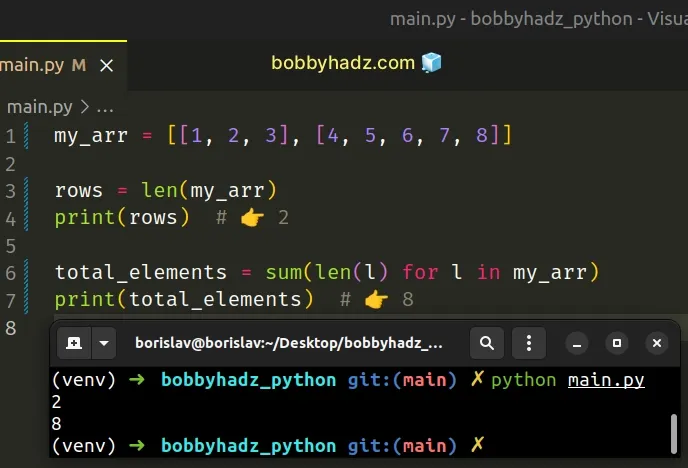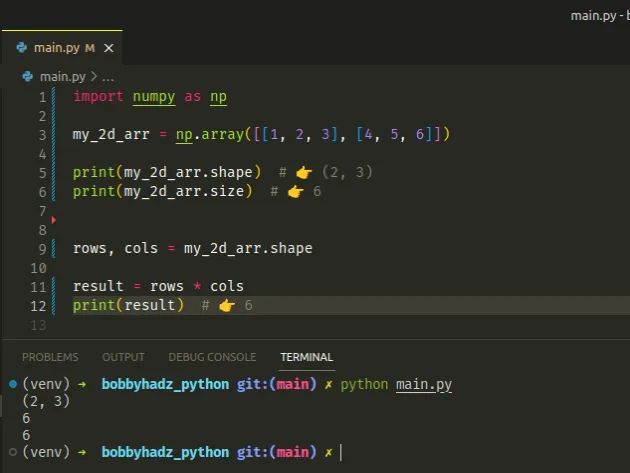# How to get the length of a 2D Array in PythonLast updated: Feb 18, 2023
4 min## #Get the length of a 2D Array in Python

To get the length of a 2D Array in Python:

1. Pass the entire array to the `len()` function to get the number of rows.
2. Pass the first array element to the `len()` function to get the number of columns.
3. Multiply the number of rows by the number of columns to get the total.
main.py
```Copied!```my_arr = [[1, 2, 3], [4, 5, 6]]

# ✅ get the number of rows
rows = len(my_arr)
print(rows)  # 👉️ 2

# ✅ get the number of columns
cols = len(my_arr)
print(cols)  # 👉️ 3

# ✅ get the number of total elements
total_elements = rows * cols
print(total_elements)  # 👉️ 6
``````The len() function returns the length (the number of items) of an object.

The argument the function takes may be a sequence (a string, tuple, list, range or bytes) or a collection (a dictionary, set, or frozen set).

We first passed the entire list to the `len()` function to get the number of rows (the number of nested lists).

main.py
```Copied!```my_arr = [[1, 2, 3], [4, 5, 6]]

rows = len(my_arr)
print(rows)  # 👉️ 2

cols = len(my_arr)
print(cols)  # 👉️ 3
``````Assuming that each nested list has the same number of elements, we can get the length of a column by accessing a nested list and passing the result to the `len()` function.

## #Getting the total number of items in a 2D list

You can get the total number of items in the 2D list by multiplying the number of rows by the number of columns.

If the nested lists may be of different sizes, use the `sum()` function.

main.py
```Copied!```my_arr = [[1, 2, 3], [4, 5, 6, 7, 8]]

rows = len(my_arr)
print(rows)  # 👉️ 2

total_elements = sum(len(l) for l in my_arr)
print(total_elements)  # 👉️ 8
``````The sum function takes an iterable, sums its items from left to right and returns the total.

We pass each nested list to the `len` function and calculate the sum.

You can also use a for loop to get the total number of items in a 2D array containing subarrays of different sizes.

main.py
```Copied!```my_arr = [[1, 2, 3], [4, 5, 6, 7, 8]]

total_elements = 0

for subarray in my_arr:
total_elements += len(subarray)

print(total_elements) # 👉️ 8
``````

We used a `for` loop to iterate over the 2D array and on each iteration, we use the add the length of the current array to the total.

## #Get the length of a 2D NumPy array

If you need to get the length of a 2D NumPy array, use the `shape` and `size` attributes.

main.py
```Copied!```import numpy as np

my_2d_arr = np.array([[1, 2, 3], [4, 5, 6]])

print(my_2d_arr.shape)  # 👉️ (2, 3)
print(my_2d_arr.size)  # 👉️ 6

rows, cols = my_2d_arr.shape

total_elements = rows * cols
print(total_elements)  # 👉️ 6
``````The size attribute returns the number of elements in the NumPy array.

main.py
```Copied!```import numpy as np

my_2d_arr = np.array([[1, 2, 3], [4, 5, 6]])

print(my_2d_arr.size)  # 👉️ 6
print(my_2d_arr.shape)  # 👉️ (2, 3)
``````

The shape attribute returns a tuple of the array's dimensions.

The first value in the tuple is the number of rows, and the second is the number of columns in the array.

The two-dimensional array contains 2 nested arrays with 3 elements each.

## #Getting the total number of elements in a NumPy 2D array

If you need to get the total number of elements in a NumPy 2D array, multiply the number of rows by the number of columns.

main.py
```Copied!```import numpy as np

my_2d_arr = np.array([[1, 2, 3], [4, 5, 6]])

print(my_2d_arr.shape)  # 👉️ (2, 3)

rows, cols = my_2d_arr.shape

total_elements = rows * cols
print(total_elements)  # 👉️ 6
``````The array in the example has 2 rows with 3 columns each, so it has a total of 6 elements.

If the nested arrays may be of different sizes, use the `sum()` function to calculate the number of total elements.

main.py
```Copied!```import numpy as np

my_2d_arr = np.array([[1, 2, 3], [4, 5, 6, 7]], dtype=object)

total_elements = sum(len(arr) for arr in my_2d_arr)

print(total_elements) # 👉️ 7
``````

On each iteration, we use the `len()` function to get the length of the current subarray and pass the result to the `sum()` function.

You can also use a simple `for` loop to calculate the number of total elements in a 2D array.

main.py
```Copied!```import numpy as np

my_2d_arr = np.array([[1, 2, 3], [4, 5, 6, 7]], dtype=object)

total_elements = 0

for arr in my_2d_arr:
total_elements += len(my_2d_arr)

print(total_elements)  # 👉️ 4
``````

On each iteration of the `for` loop, we add the length of the current array to the `total_elements` variable.

I've also written an article on how to check if a value exists in a two-dimensional list.

I wrote a book in which I share everything I know about how to become a better, more efficient programmer.You can use the search field on my Home Page to filter through all of my articles.